# Interpretability and Explainability for Credit Scoring

This example shows different techniques for interpreting and explaining the logic behind credit scoring predictions.

While credit scorecard models, in general, are straightforward to interpret, this example uses a black-box model, without revealing the logic, to show the workflow for explaining predictions. In this example, you work with the `creditscorecard` object from Financial Toolbox™ and pass the scoring function to interpretability tools in Statistics and Machine Learning Toolbox™. These tools include:

These tools support regression and classification modeling, which make interpretation more efficient. For more information on these techniques, see Interpret Machine Learning Models. In this example, the score model of the `creditscorecard` object is used as the black-box model. For an example of this workflow, see Interpret and Stress-Test Deep Learning Networks for Probability of Default.

### Background

Credit scoring is the process by which lenders assign scores to borrowers and use those scores to decide whether or not to accept a loan application. Lenders use credit scoring models to come up with these scores. Traditionally, simple, interpretable models such as credit scorecards and logistic regression have been widely used in this area. Over time, Machine Learning (ML) and Artificial Intelligence (AI) techniques were introduced to implement credit scoring models. Such techniques, while improving predictive power, also are more black-box and there is little or no explanation behind the decisions. Consequently, the credit scoring predictions of ML and AI techniques are difficult for humans to interpret. As a result, lenders are implementing different interpretability and explainability methods to get a better understanding of the logic behind the credit scoring predictions. In addition, regulators are also requiring that practitioners use more interpretability and also fairness methods to ensure that no equal opportunity laws are broken while making credit decisions. For more information on using fairness metrics, see Explore Fairness Metrics for Credit Scoring Model.

### Create Credit Scorecard Model

Load credit card data and create a credit scorecard model using the `creditscorecard` object.

```load CreditCardData sc = creditscorecard(data,IDVar="CustID");```

Apply automatic binning. This example uses a split algorithm, with a maximum of 5 bins per predictor and with the constraint that each bin has at least 50 observations. For more information, see `autobinning` and Bin Data to Create Credit Scorecards Using Binning Explorer.

`sc = autobinning(sc,Algorithm="Split",AlgorithmOptions={"MaxNumBins",5,"MinCount",50});`

Verify that the binning for the numeric variables has five bins or levels. For example, here is the bin information for the customer age predictor.

```bi = bininfo(sc,"CustAge"); disp(bi)```
``` Bin Good Bad Odds WOE InfoValue _____________ ____ ___ ______ _________ __________ {'[-Inf,35)'} 93 76 1.2237 -0.50255 0.038003 {'[35,47)' } 321 184 1.7446 -0.14791 0.0094258 {'[47,53)' } 194 64 3.0312 0.40456 0.03252 {'[53,61)' } 128 64 2 -0.011271 2.0365e-05 {'[61,Inf]' } 67 9 7.4444 1.303 0.079183 {'Totals' } 803 397 2.0227 NaN 0.15915 ```

For categorical variables, there may be fewer than five bins in total, since the split algorithm in `autobinning` can merge categories into a single group. For example, the residential status predictor initially has three levels: Tenant, Home Owner, and Other. The split algorithm returns only two groups.

```[bi,cg] = bininfo(sc,"ResStatus"); disp(bi)```
``` Bin Good Bad Odds WOE InfoValue __________ ____ ___ ______ _________ _________ {'Group1'} 672 344 1.9535 -0.034802 0.0010314 {'Group2'} 131 53 2.4717 0.20049 0.0059418 {'Totals'} 803 397 2.0227 NaN 0.0069732 ```

The category grouping information shows that Tentant and Home Owner are merged into `Group1`. This grouping means Tenant and Home Owner will get the same number of points in the final scorecard.

`disp(cg)`
``` Category BinNumber ______________ _________ {'Tenant' } 1 {'Home Owner'} 1 {'Other' } 2 ```

Fit the model coefficients using `fitmodel`. For illustration purposes, keep only five model predictors, including some categorical ones.

```PredictorsInModel = ["CustAge" "CustIncome" "EmpStatus" "ResStatus" "UtilRate"]; sc = fitmodel(sc,PredictorVars=PredictorsInModel,VariableSelection="fullmodel",Display="off");```

Scale the points so that `500` points correspond to odds of `2`, and the odds double every `50` points.

`sc = formatpoints(sc,PointsOddsAndPDO=[500 2 50]);`

Display the scorecard using `displaypoints`. The credit scorecard model is a lookup table. For example, for customer age there are five bins or levels with different points for each level. A visualization of the score, as a function of age, has a piecewise constant pattern with five levels, as shown in Partial Dependence Plot. For residential status, Tenant and Home Owner are in `Group1`, and they get the same number of points.

```[ScorecardPointsTable,MinPts,MaxPts] = displaypoints(sc); disp(ScorecardPointsTable)```
``` Predictors Bin Points ______________ _________________ ______ {'CustAge' } {'[-Inf,35)' } 71.84 {'CustAge' } {'[35,47)' } 91.814 {'CustAge' } {'[47,53)' } 122.93 {'CustAge' } {'[53,61)' } 99.511 {'CustAge' } {'[61,Inf]' } 173.54 {'CustAge' } {'<missing>' } NaN {'ResStatus' } {'Group1' } 97.318 {'ResStatus' } {'Group2' } 116.43 {'ResStatus' } {'<missing>' } NaN {'EmpStatus' } {'Unknown' } 85.326 {'EmpStatus' } {'Employed' } 118.11 {'EmpStatus' } {'<missing>' } NaN {'CustIncome'} {'[-Inf,31000)' } 68.158 {'CustIncome'} {'[31000,38000)'} 102.11 {'CustIncome'} {'[38000,42000)'} 93.302 {'CustIncome'} {'[42000,47000)'} 109.18 {'CustIncome'} {'[47000,Inf]' } 121.21 {'CustIncome'} {'<missing>' } NaN {'UtilRate' } {'[-Inf,0.12)' } 106.84 {'UtilRate' } {'[0.12,0.3)' } 94.647 {'UtilRate' } {'[0.3,0.39)' } 140.95 {'UtilRate' } {'[0.39,0.68)' } 69.635 {'UtilRate' } {'[0.68,Inf]' } 94.634 {'UtilRate' } {'<missing>' } NaN ```

One "traditional" approach to measure the importance of each predictor in the credit scorecard model is to compute the percent of the total score range that comes from each predictor.

```PtsRange = MaxPts - MinPts; NumPred = length(PredictorsInModel); PercentWeight = zeros(NumPred,1); for ii = 1 : NumPred Ind = strcmpi(PredictorsInModel{ii},ScorecardPointsTable.Predictors); MaxPtsPred = max(ScorecardPointsTable.Points(Ind)); MinPtsPred = min(ScorecardPointsTable.Points(Ind)); PercentWeight(ii) = 100*(MaxPtsPred-MinPtsPred)/PtsRange; end PredictorWeights = table(PredictorsInModel',PercentWeight,VariableNames=["Predictor" "Weight"]); disp(PredictorWeights)```
``` Predictor Weight ____________ ______ "CustAge" 36.587 "CustIncome" 19.085 "EmpStatus" 11.795 "ResStatus" 6.8768 "UtilRate" 25.656 ```

Customer age is the main variable in the model, since it corresponds to 36% of the total score range. A customer can get anywhere from `71.8` to `173.5` points, based on their age. This range has a difference of over 100 points between the minimum and maximum values. On the other end, residential status plays a minor role in the score, with points ranging from `97.3` to `116.4` only, a difference of less than 20 points.

An alternative to this "traditional" approach is to use the following explainability techniques from Statistics and Machine Learning Toolbox: Partial Dependence Plot, Individual Conditional Expectation Plot, Local Interpretable Model-Agnostic Explanation Plot, and Shapley Values.

### Partial Dependence Plot

The partial dependence plot (PDP) shows the effect of one or two variables on the predicted score.

Use the `plotPartialDependence` function to pass the `score` method of the `creditscorecard` object as a black-box model.

#### One Predictor

Select a predictor using the dropdown option.

As an example, if customer age is selected, note the piecewise constant shape of the plot, with jumps occuring at the bin edges, and with five levels in total. This is consistent with the five bins for customer age in the credit scorecard model.

```predictor =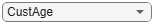PredictorsInModel(1); plotPartialDependence(@(tbl)score(sc,tbl),predictor,data)```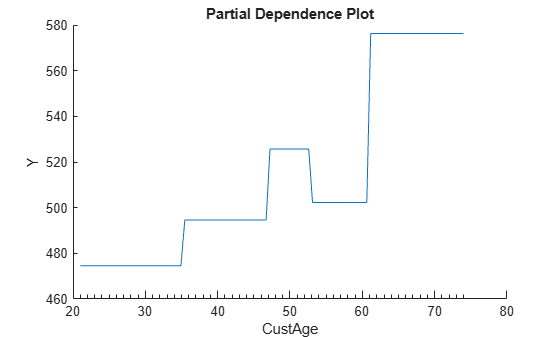#### Two Predictors

Generating a partial dependence plot with two predictors can take significantly longer than the one-predictor case. Typically, the more unique values a predictor has in the data set, the longer it takes to plot the partial dependence. Here's a report of the number of unique values in the data.

```NumUniqueValuesTable = varfun(@(x)length(unique(x)),data(:,PredictorsInModel)); NumUniqueValuesTable.Properties.VariableNames = erase(NumUniqueValuesTable.Properties.VariableNames,'Fun_'); disp(NumUniqueValuesTable)```
``` CustAge CustIncome EmpStatus ResStatus UtilRate _______ __________ _________ _________ ________ 54 45 2 3 110 ```

The categorical predictors have fewer unique levels, so these plots for categorical predictors run faster. Numeric variables like customer age are relatively discrete and so is utilization rate because this rate's values are rounded to two decimals. However, a continuous predictor (for example, the average monthly balance (`AMBalance`) in the `data` table) can have many unique values.

Select a predictor and additional predictor to then use `plotPartialDependence` to generate the PDP plot.

```predictor =PredictorsInModel(1); additionalPredictor =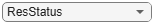PredictorsInModel(4); plotPartialDependence(@(tbl)score(sc,tbl),[predictor,additionalPredictor],data)```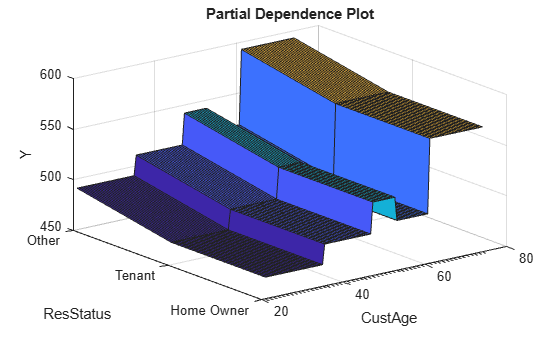### Individual Conditional Expectation Plot

Similar to the partial dependence plot, the individual conditional expectation plot (ICE) shows the effect of one of the variables on the predicted score. The red line in the ICE plot matches the partial dependence plot. While the partial dependence plot shows the average score as a function of the selected predictor, the ICE plot disaggregates and shows the score for each observation (each gray line) as a function of the selected predictor. For more information, see the More About section on the `plotPartialDependence` reference page.

```predictor =PredictorsInModel(1); plotPartialDependence(@(tbl)score(sc,tbl),predictor, ... data,Conditional="absolute")```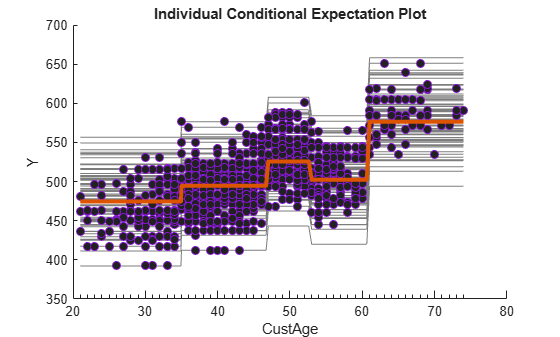### Select a Query Point

The PDP and ICE plots provide a global view of the credit scorecard scores, where the score is visualized for all values of the selected predictor. In contrast, LIME and Shapley are local explainability techniques that explain the behavior of the model in a neighborhood of a query point of choice. For more information, see Interpret Machine Learning Models.

To see how a query point helps to explain credit scores , use index 92 in the training data as your query point. You can select other query points by typing an index value into the text box.

`QueryPointIndex =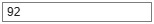92; % ID number of the observation to explain`

Use `score` to display the query point score and the points, by predictor, for this query point.

```[ScoresTraining,PointsTraining] = score(sc,data); fprintf("Selected index %d, with score %g\n",QueryPointIndex,ScoresTraining(QueryPointIndex))```
```Selected index 92, with score 417.289 ```
`disp(PointsTraining(QueryPointIndex,:))`
``` CustAge ResStatus EmpStatus CustIncome UtilRate _______ _________ _________ __________ ________ 71.84 97.318 85.326 68.158 94.647 ```

The plots that follow show the location of the query point (dotted vertical line) relative to the distribution of values for the scores and for each predictor. For example, for index `92`, the score is low relative to the distribution. For the customer age predictor, the query point is on the bottom group. This result is similar for the customer income, employment status, and residential status predictors. The points for the utilization rate predictor are closer to the middle of the distribution, but still below average.

```figure t = tiledlayout(3,2); nexttile plotQueryInHistogram("Score",QueryPointIndex,ScoresTraining,PointsTraining) nexttile plotQueryInHistogram("CustAge",QueryPointIndex,ScoresTraining,PointsTraining) nexttile plotQueryInHistogram("CustIncome",QueryPointIndex,ScoresTraining,PointsTraining) nexttile plotQueryInHistogram("EmpStatus",QueryPointIndex,ScoresTraining,PointsTraining) nexttile plotQueryInHistogram("ResStatus",QueryPointIndex,ScoresTraining,PointsTraining) nexttile plotQueryInHistogram("UtilRate",QueryPointIndex,ScoresTraining,PointsTraining) title(t,"Query Point Relative to Distribution")```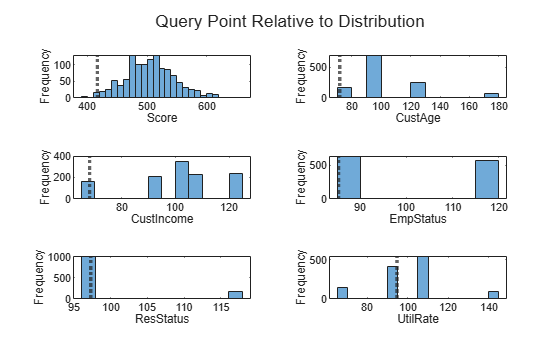### Local Interpretable Model-Agnostic Explanation Plot

The local interpretable model-agnostic explanation (LIME) plot shows the coefficients of a local linear model near the instance of a score that you want to explain. LIME explains the scores around a particular observation, or query point, with a simple local model, such as a linear regression model or a decision tree.

Use `lime` to create a `lime` object specifying the data set of interest (the training data set), the model "type" (use "regression" to indicate a numeric prediction), and which variables are categorical. When you create a `lime` object, the toolbox generates a random synthetic data set. Use the synthetic data to fit simple local models to explain the local behavior.

```rng('default'); % for reproducibility limeExplainer = lime(@(tbl)score(sc,tbl),data(:,PredictorsInModel),Type="regression", ... CategoricalPredictors=["ResStatus" "EmpStatus"]);```

Select a maximum number of predictors (`NumPredToExplain`) to explain and use a `SimpleModelType` of a `"tree"` to explain the local behavior of the score. The results are sensitive to the kernel width parameter (`KernelWidthChoice`) that controls how much neighbor points are weighted while fitting the linear simple model.

```NumPredToExplain =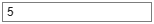5; % number of variables/predictors to explain KernelWidthChoice =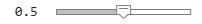0.5; limeExplainer = fit(limeExplainer,data(QueryPointIndex,PredictorsInModel), ... NumPredToExplain,SimpleModelType="tree",KernelWidth=KernelWidthChoice); figure f = plot(limeExplainer);```When the simple model is a tree, based on the reported predictor importance, customer age is the main predictor, followed by employment status and customer income.

### Shapley Values

Shapley values explain the deviation of the predicted score from the average predicted score. The sum of the Shapley values for all predictors corresponds to the total deviation of the score for the query point from the average score.

The Shapley values are estimated based on a simulation. For larger data sets, this simulation is time consuming. For illustration purposes, this example uses only 500 rows of the training data with the `shapley` constructor.

```rng('default'); % for reproducibility shapleyExplainer = shapley(@(tbl)score(sc,tbl),data(500,PredictorsInModel), ... QueryPoint=data(QueryPointIndex,PredictorsInModel),CategoricalPredictors=["EmpStatus" "ResStatus"]); figure plot(shapleyExplainer)```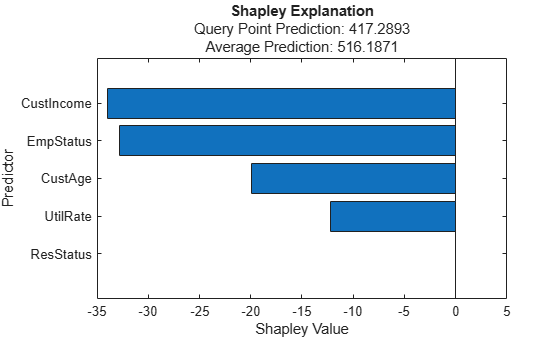For the query point with index `92`, the predicted score is `417`, whereas the average score for the training data set passed to `shapley` function is `516`. You expect the Shapley values to be negative, or at least have important negative components that explain why the predicted score is below average. In contrast, for scores above average, the Shapley values add up to a positive amount. In this example, the estimated Shapley values show that the main deviation from the average is explained by the customer income and employment status predictors, followed by the customer age and utilization rate predictors. The residential status predictor is not important. This result might be a combination of the simulation itself with the fact that residential status has a smaller impact on scores for this model.

### Final Remarks

Explainability techniques are widely used to understand the behavior of predictive models. In this example, a `creditscorecard` model shows how explainability techniques, such as PDP, ICE, LIME, and Shapley are applied to explain a black-box model. Although credit scorecard models are simple and interpretable, you can apply the explainability tools in this example to other scoring models that are treated as black-box models or to supported models in Statistics and Machine Learning Toolbox. Alternatively, instead of explaining the scores, you can pass the `probdefault` function as the black-box model to explain the probability of default predictions.

### Local Functions

```function plotQueryInHistogram(VariableChoice,QueryPointIndex,Scores,PointsTable) if VariableChoice=="Score" HistData = Scores; else HistData = PointsTable.(VariableChoice); end histogram(HistData) hold on xline(HistData(QueryPointIndex),':','LineWidth',2.5) hold off xlabel(VariableChoice) ylabel('Frequency') end```